# Java QuickSort Example | QuickSort In Java Program

Java Quicksort is thought to be the fastest sorting algorithm. The time complexity in quicksort is O(n log n) for the best and average case and O(n^2) in the bad case. And as the time complexity is the biggest thing that should be kept in the mind, so we always preferred quicksort in many cases among any other sorting algorithm.

## Java QuickSort Example

QuickSort is the Divide and Conquer algorithm. It picks an item as a pivot element and partitions the given array around the selected pivot. There are many different versions of the quickSort that pick pivot in different ways.

1. Always pick the first element as a pivot.
2. Always pick the last item as the pivot (implemented below)
3. Pick a random item as the pivot.
4. Pick median as the pivot.

The key process in quickSort is a partition(). The target of partitions is, given an array and an element x of an array as the pivot, put x at its correct position in a sorted array and put all smaller elements (smaller than x) before x, and put all greater items (greater than x) after x. All this should be done in linear time.

## #Pseudo Code for Quick Sort

```QUICKSORT(A, start, end)

1. if start < end
2. P_indx =PARTITION(A, start, end)
3. QUICKSORT(A, start, P_indx-1)
4. QUICKSORT(A, P_indx +1, end)
5. Exit

PARTITION(A, start, end)
1. Set pivot = A[end]
2. Set P_indx = start
3. Repeat step 4 to 7 for j = start to end - 1
4. if A[j]<=pivot
5. exchange A[P_index] with A[j]
6. Set P_index=P_index+1
[End of If block]
7. exchange A[P_index] with A[end]
[End of For Loop]
8. return P_index```

## #Let’s see what Quicksort is

In quicksort, we have first to choose a pivot point in the given array which we have to sort. And then on the left side, we have to keep all the items less than the pivot, and the numbers greater than the pivot are kept on the right side.

And then in left and right of the pivot, we have to do the same thing recursively. And in the end, we will be able to get a sorted array.

Now the question arises that how to choose the pivot point?

The pivot point can be any of the given element. Maybe the first one, the last one, the middle one or any random number.

Now let’s clear the topic through examples.

### #Step 1: By taking the first element as a pivot point

Suppose we have an array ->  30, 20, 5, 60, 22, 91

#Step1: Select the pivot point.

Our pivot point is 30 in this case.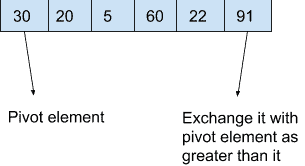#Step2: Now we have to arrange numbers smaller than the pivot point in the left and greater than the pivot point in the right.

First of all, take the pivot to the end.

Swap 30 and 91 { 91, 20, 5, 60, 22, 30}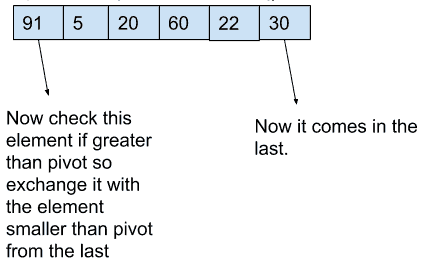Then check 91, as it is greater than 30 so check with last-second that is 22 which is less than pivot so swap 91 and 22{ 22, 20, 5, 60, 91, 30}

Then check 22 and 60 as 22 is not greater than 30, no swapping should be done.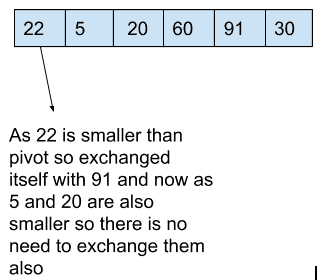Then check 20 and 5 are also not greater than 30 so not swapping and

As 60 is greater than 30 so swap 60 with 30 which will arrange array as:

{ 22, 20, 5, 30, 91, 60}.

This is the first partitioning we have performed successfully.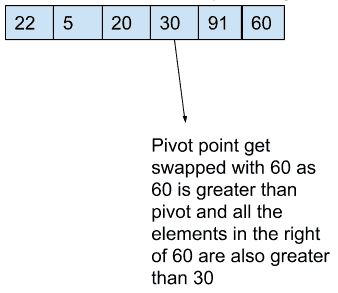Step3: Now do the same process with the left and right of the pivot point.

Left part : { 22, 20, 5}                      Right part : { 91, 60}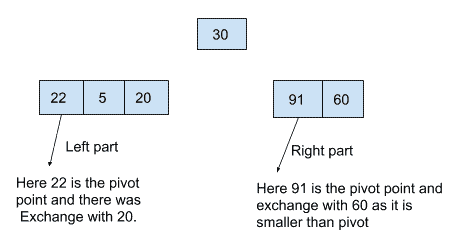On the left part choose 22 as the pivot point So swap 22 with 5. This would become { 5, 20,22}.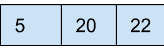And then also choose left of pivot: { 5, 20}.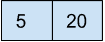In the right part choose 91 as the pivot and then swap 91 and 60.{ 60, 91}.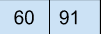And then merge the whole array as:

{5, 20, 22, 30, 60, 91}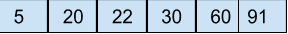This is the sorted array.

### #Step: 2 By taking the last element as the pivot element.

Suppose we have an array ->  30, 20, 5, 60, 22, 91

Step1: Select the pivot point.

Our pivot point is 91 in this case.

Step2: Now we have to arrange numbers smaller than the pivot point in the left and greater than the pivot point in the right.

In this there is no need to take the element in the last but the work we have to do is to check the starting elements and compare one by one with the last element if it was smaller than pivot we have to do nothing but if it was larger than the pivot then swap that element with the next smaller one in that row.

Almost the procedure is the same in both cases.

So, let’s see the example by taking the last element as the pivot element.

### #Programming Example

See the following program.

```class Sort {
public int partition(int ar[], int start, int end) {
int pivot = ar[end];
int i = (start - 1);
for (int j = start; j < end; j++) {
if (ar[j] <= pivot) {
i++;
int temp = ar[i];
ar[i] = ar[j];
ar[j] = temp;
}
}
int temp = ar[i + 1];
ar[i + 1] = ar[end];
ar[end] = temp;
return (i + 1);
}

void sort(int ar[], int start, int end) {
if (start < end) {
int p = partition(ar, start, end);
sort(ar, start, p - 1);
sort(ar, p + 1, end);
}
}

public static void main(String args[]) {
int ar[] = { 30, 20, 5, 60, 22, 91 };
int n = ar.length;
Sort s = new Sort();
s.sort(ar, 0, n - 1);
System.out.println("Array after Sorting is: ");
for (int i = 0; i < n; ++i)
System.out.print(ar[i] + " ");
System.out.println();
}
}```

See the following output.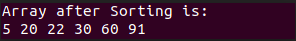Finally, Java QuickSort Program is over.

This site uses Akismet to reduce spam. Learn how your comment data is processed.